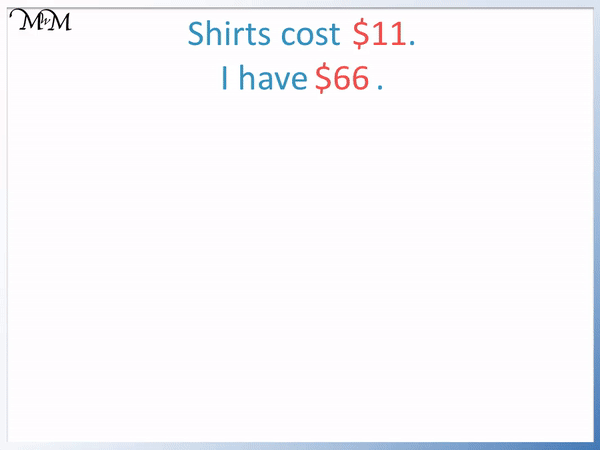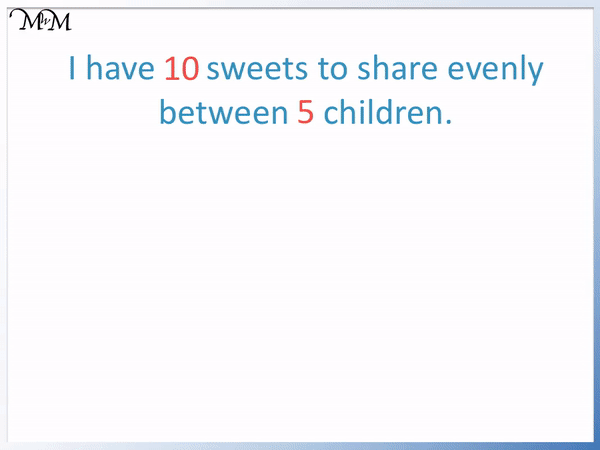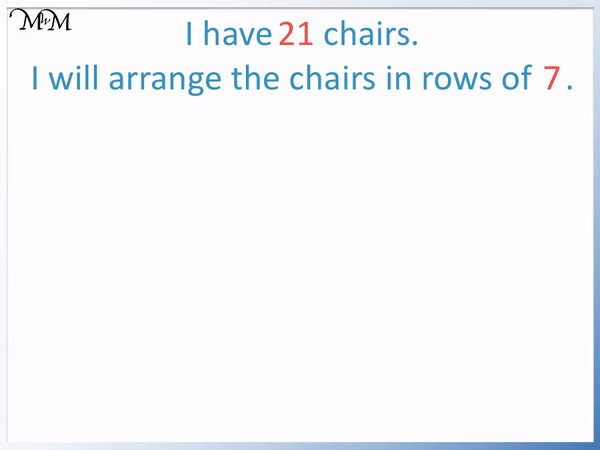# Division Word Problems

Division Word Problems• I have 80 matches and I will put 8 into each packet.
• There are two numbers in this question: 80 and 8.
• We identify the total number, which is 80.
• We identify the number in each group, which is 8.
• We divide the total by the number in each group to find the number of groups.
• 80 ÷ 8 = 10 and so, we can make 10 packets.

Divide the total by the number of groups to find the amount in each group.

Divide the total by the amount in each group to find the number of groups.• Each shirt costs $11 and I have$66.
• We identify $66 as the total. •$11 is the cost of each shirt.
• We want to know how many times 11 can go into 66.
• 66 ÷ 11 = 6 and so, 11 goes into 66 six times.
• We can spend $11 six times.Supporting Lessons#### Simple Division Word Problems: Interactive Questions #### Simple Division Word Problems Decimals Worksheets and Answers # Division Word Problems ## How to Identify Division Word Problems When an amount is shared into equal parts and we are asked for how many parts, or the number in each part, then we have a division. To identify a division word problem, we need to read the question carefully and try to understand the context. If we have been told the total and are sharing this total into equally sized parts, then a division is taking place. Division keywords can also help to give us a clue that we have a division. Here are some division keywords: • Share • Between • Evenly • Equally • Per • Shared • Split However it is always worth reading the question carefully to make sure that we are sharing a total into an equally sized parts. ## Simple Division Word Problems To solve a division word problem, we can use the following steps: 1. Identify the numbers given in the question. 2. Identify which number is the total quantity. 3. Identify how many groups we are sharing between or how many need to go in each group. 4. Divide the total by the number of groups to find the amount in each group. 5. Or divide the total by how many needed in each group to find out how many groups can be made. 6. In this example, ‘I have 10 sweets to share evenly between 5 children. How many sweets do they each get?’ We are trying to find how many sweets each child gets, so we want to know how many sweets will be in each group. We first identify the total, which is 10 sweets. Now we identify how many groups we have, which is 5. We are sharing equally between 5 children. We divide the total amount by the number of groups.We are sharing 10 sweets between 5 people. 10 ÷ 5 = 2 and so, each child gets 2 sweets each. When teaching division word problems, we can draw 10 sweets and group them equally by drawing circles around them to help visualise this. We could also get 10 counters and share them out equally, one at a time. We can see in this example that we had the keywords of share evenly and each, which can give us a clue that we have a division. The division also tells us how many times 5 goes into 10.In this next example, ‘I have 80 matches. I will put 8 into each packet. How any packets will I fill?’ We want to see how many packets we will fill. We want to see how many groups we will create. We first identify the total number of matches, which is 80. We then identify the number in each packet, which is 8. To find the total number of packets, we will divide.80 ÷ 8 = 10 and so, we can make 10 packets. We can think of this as working out how many times 8 goes into 80 or how many packets can be made from 80 matches. In this next example, ‘I need 30 crayons. Each pack contains 5 crayons. How many packs should I buy?’ The total number is the larger number, which is is 30. We are buying the crayons in equal groups of 5. We need to work out how many groups we need. How many fives make 30? We need to work out how many fives go into 30.30 ÷ 5 = 6 and so, we need 6 packs. We can check out answer. 6 lots of 5 make the 30 crayons needed because 6 × 5 = 30. In this example we needed to find the number of groups required. So we divided the total by the number in each group. In this example, ‘I have 21 chairs. I will arrange the chairs in rows of 7.How many rows should I make?’ Here we have the total number of chairs, which is 21. We are arranging them into rows of 7, so each group contains 7 chairs. We want to find the number of rows that we can make. We want to work out how many rows of 7 can be made from 21 chairs. This is how many times 7 goes into 21.21 ÷ 7 = 3 and so, we can make 3 rows. We can see that each row is the same size. We can teach this by taking 21 counters and sharing them into 3 equal rows. In this example involving money, ‘Shirts cost$11 and I have $66. How many shirts can I buy?’ We want to know how many elevens go into 66. The total is$66 and we are dividing by 11.We want to know how many times we can spend $11. 66 ÷ 11 = 6 and so, we can spend$11 six times.